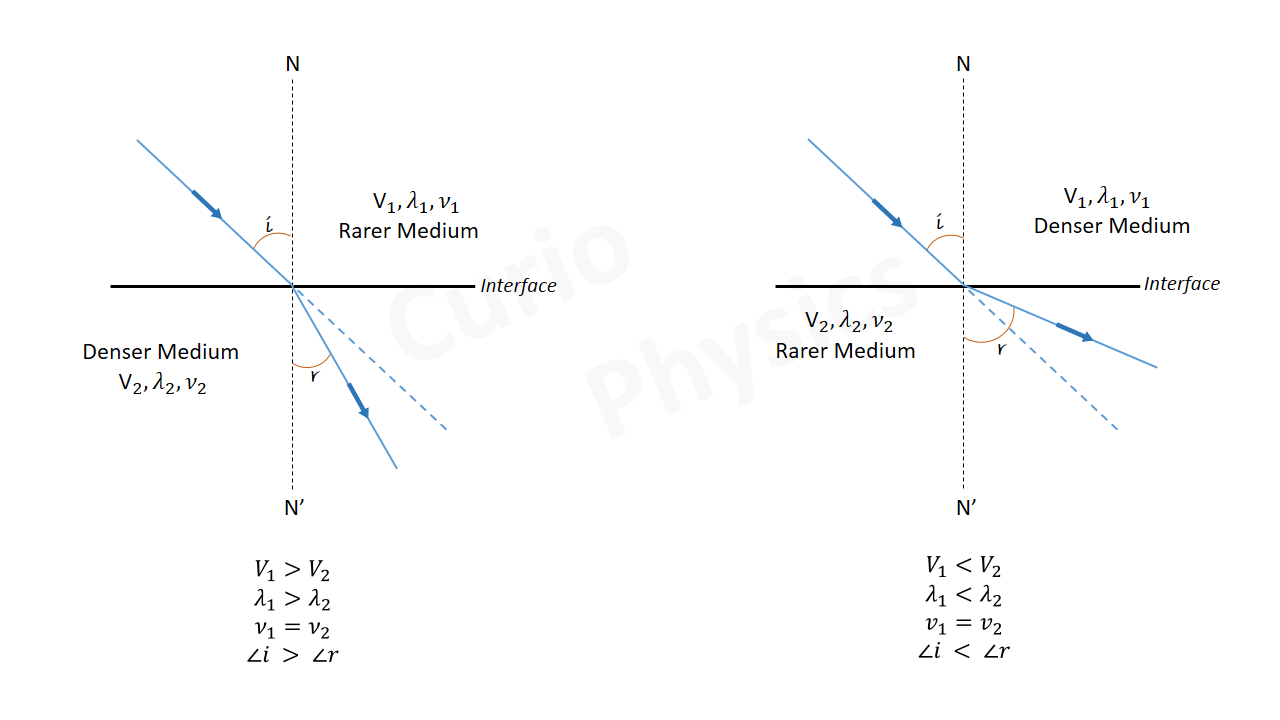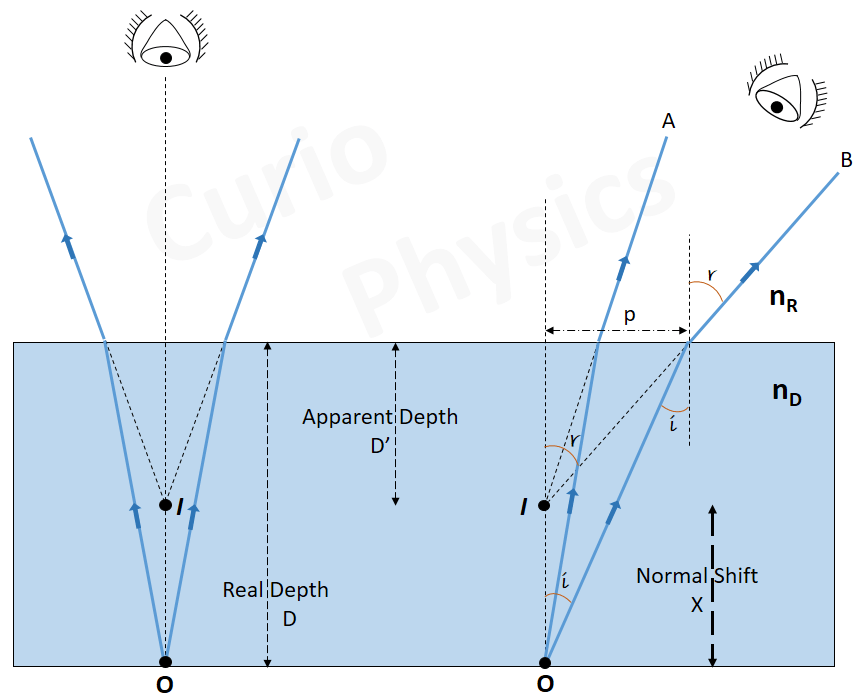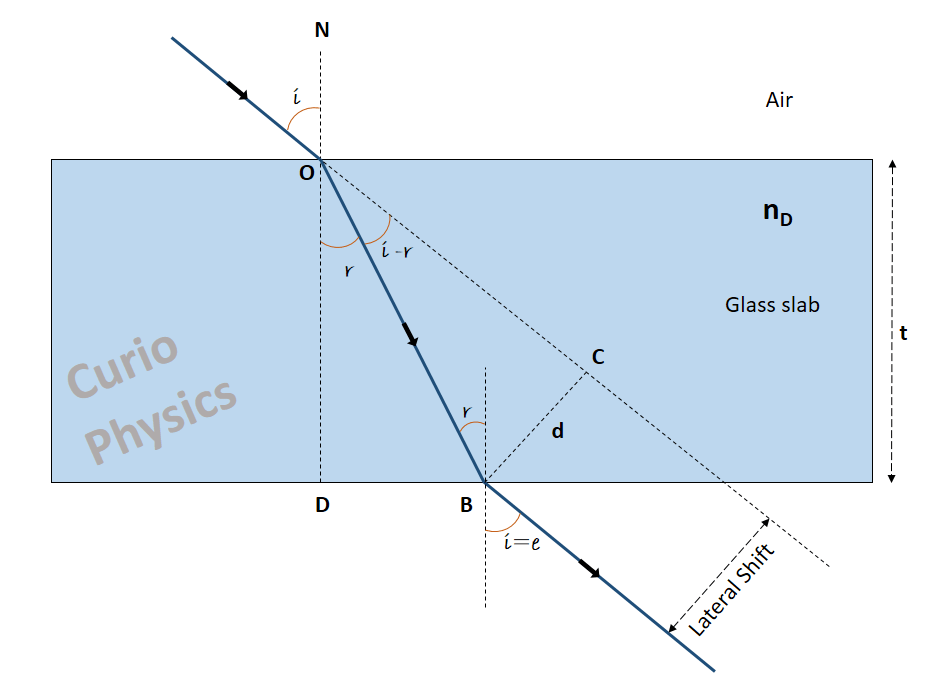# Refraction of light

Refraction of light:- When a ray of light enters from one medium to another transparent medium, a part of the light gets reflected back in the first medium while the remaining part enters the transparent medium. This remaining part, which enters the other medium, changes its direction of propagation at the boundary (interface). This phenomenon is called refraction of light.

Hence, refraction is the phenomenon in which the direction of propagation of light changes at the interface when it passes from one medium to another. Not only light but other waves, such as sound waves and water waves also experience refraction.

The working of optical instruments such as magnifying glasses, lenses, prisms and human eye(to focus light on to our retina) is based on refraction of light.

During refraction of light, the speed and wavelength changes but frequency remains the same. The reason of refraction(bending of light), is the change in speed of light while going from one medium to another.From above figure, we observe that as the light ray goes from rarer to denser medium, it bends towards the normal and when the light ray goes from denser to rarer, then it bends away from the normal.

#### Snell’s Law(Refraction of light) :-

Snell experimentally obtained the following laws of refraction :-
(i) The incident ray, the refracted ray and the normal to the interface at the point of incidence, all lie in the same plane.
(ii) The ratio of the sine of the angle of incidence, to the sine of angle of refraction is constant for a given pair of media, i.e.,$\displaystyle \frac{\sin i}{\sin r}={{n}_{21}}$

Remember that the angles of incidence (i) and refraction (r) are the angles that the incident and its refracted ray make with the normal, respectively.

n21 is called the refractive index of the second medium with respect to the first medium.  Refractive index is a characteristic of the pair of media
(and also depends on the wavelength of light), but is independent of the angle of incidence.

From above equation, if n21 > 1, then r < i, i.e., the refracted ray bends towards
the normal. In this case the medium in which the light ray enters is said to be optically denser than the medium from which light comes.

On the other hand, if n21 < 1, r > i, i.e., the refracted ray bends away from the normal. This is the case when incident ray in a denser medium refracts into a rarer medium.

Note :- Optical density is different from mass density(mass per unit volume). Optical density is related to speed of light. The medium in which speed of light is more is considered as rarer medium and the medium in which speed of light is less is considered as denser medium.

It is possible that mass density of an optically denser medium may be less than that of an optically rarer medium. For example, mass density of turpentine is less than that of water but its optical density is higher.

Absolute refractive index :-

It is defined as the ratio of speed of light in free space ‘c’ to that in a given medium ‘v’, i.e., n = c/v

Denser is the medium, lesser will be the speed of light and so greater will be the refractive index,

Vglass  <  Vwater  ⇒  nG > nW

Relative refractive index :-

When light passes from one medium to the other, the refractive index of medium 2 relative to 1 is written as n21 and is defined as$\displaystyle {{n}_{21}}=\frac{{{n}_{2}}}{{{n}_{1}}}=\frac{(c/{{v}_{2}})}{(c/{{v}_{1}})}=\frac{{{v}_{1}}}{{{v}_{2}}}$Note :-

(1). If n21 is the refractive index of medium 2 with respect to medium 1 and n12 the refractive index of medium 1 with respect to medium 2, then it should be clear that$\displaystyle {{n}_{12}}=\frac{1}{{{n}_{21}}}$

(2). If n32 is the refractive index of medium 3 with respect to medium 2 then n32 = n31 × n12, where n31 is the refractive index of medium 3 with respect to medium 1.

[$\displaystyle {{n}_{32}}=\frac{{{n}_{31}}}{{{n}_{21}}}\Rightarrow {{n}_{32}}=\left( \frac{{{n}_{31}}}{\frac{1}{{{n}_{12}}}} \right)\Rightarrow {{n}_{32}}={{n}_{31}}\times {{n}_{12}}$]

##### Natural Phenomenon due to Refraction of Light :-

(a) The Twinkling Stars :- Light from the stars travel in straight lines through the vacuum of space. When these light rays enter the Earth’s atmosphere, they are refracted by layers of atmosphere of different densities. The densities of these layers of atmosphere are constantly changing (due to wind and temperature), thus altering the path of the light rays. One moment the light rays are refracted in such a way that it reaches your eyes and the next moment they are not. This produces the perception that the stars are twinkling. If one were to observe the same stars from space, they would not twinkle at all.

(b) Bottom of a tank filled with water appears to be raised :-

If a point object is in denser medium and is observed from rarer medium and the boundary is plane, then the object(O) appears to be raised(Image I), as shown in figure below :-From Snell’s law we have$\displaystyle \frac{\sin i}{\sin r}={{n}_{RD}}$

But as$\displaystyle {{n}_{21}}=\frac{{{n}_{2}}}{{{n}_{1}}}$

So$\displaystyle \frac{\sin i}{\sin r}=\frac{{{n}_{R}}}{{{n}_{D}}}$$\displaystyle \Rightarrow {{n}_{D}}\sin i={{n}_{R}}\sin r$     …..(1)

If the rays OA and OB are close enough to reach the eye, then angles i and r will be small,$\displaystyle \sin i\approx \tan i=\frac{p}{D}$

and$\displaystyle \sin \text{r}\approx \tan \text{r}=\frac{p}{D'}$

Now the equation (1) becomes$\displaystyle {{n}_{D}}\frac{p}{D}={{n}_{R}}\frac{p}{D'}$$\displaystyle \frac{D}{D'}=\frac{{{n}_{D}}}{{{n}_{R}}}$

Now if we take nR = 1(refractive index of air) and nD as n(refractive index of denser medium), then$\displaystyle D'=\frac{D}{n}$     …..(2)

(⇒ D’ < D, which is clear from the figure)

Now the distance between object and its image, called normal shift (x), which is given by

x = D – D’

Now as$\displaystyle D'=\frac{D}{n}$, from equation (2), hence$\displaystyle x=D-D'=D-\frac{D}{n}$$\displaystyle \Rightarrow x=D\left[ 1-\frac{1}{n} \right]$     …..(3)

(c) Object in a rarer medium is seen from a denser medium(A Fish Eye View) :-

A fish or a diver can see objects above the water surface, but the objects in the air would appear to be raised up at a different positions as shown in figure below :-From Snell’s Law,$\displaystyle \frac{Sini}{Sinr}=\frac{{{n}_{D}}}{{{n}_{R}}}$     …..(4)

if angles i and r , are small, then$\displaystyle \sin i\approx \tan i=\frac{AB}{h}$

and$\displaystyle \sin \text{r}\approx \tan \text{r}=\frac{AB}{h'}$

From equation (4),$\displaystyle \frac{\frac{AB}{h}}{\frac{AB}{h'}}=\frac{{{n}_{D}}}{{{n}_{R}}}$$\displaystyle \Rightarrow \frac{h'}{h}=\frac{{{n}_{D}}}{{{n}_{R}}}$

Now if we take nR = 1(refractive index of air) and nD as n(refractive index of denser medium), then$\displaystyle h'=nh$

(i.e., h’ > h)

Shift x = h’ – h = nh – h = h(n-1)     …..(5)

(d) Lateral Shift :-

For a rectangular slab, refraction takes place at two interfaces (air-glass and glass-air). It is easily seen from below figure, that the angle of emergence = angle of incidence, i.e., i = e. The emergent ray is parallel to the incident ray—there is no deviation, but it does suffer lateral displacement/ shift with respect to the incident ray.Lateral displacement/ shift :- The perpendicular distance between incident and emergent ray is known as lateral shift.

Lateral shift d = BC and t = thickness of slab

In ΔBOC :-$\displaystyle \sin (i-r)=\frac{BC}{OB}=\frac{d}{OB}$$\displaystyle \Rightarrow d=OB\sin (i-r)$     …..(6)

In ΔOBD :-$\displaystyle cos\text{r}=\frac{OD}{OB}=\frac{t}{OB}$$\displaystyle \Rightarrow OB=\frac{t}{Cosr}$     …..(7)

From (6) and (7),$\displaystyle d=\frac{t}{Cosr}\sin (i-r)$     …..(8)

(e) Atmospheric Refraction of Light :-

The sun is visible a little before the actual sunrise and until a little after the actual sunset due to refraction of light through the atmosphere.  Actual sunrise mean the time when sun actually cross the horizon. Below figure shows the actual and apparent positions of the sun with respect to the horizon.(Image above is highly exaggerated)

The refractive index of air with respect to vacuum is 1.00029. This means that the speed of light in air is 1.00029 times slower than the speed of light in vacuum. This causes the light rays to bend towards the normal. Due to this, the apparent shift in the direction of the sun is by about 0.5° and the sunrise happens 2 minutes before actual sunrise and sunset happens 2 minutes after the actual sunset, thereby lengthening the day by 4 minutes.

The apparent flattening (oval shape) of the sun at sunset and sunrise is also due to the same phenomenon. Objects closer to the horizon are raised upwards. Due to this the lower limb of the sun is raised more than the top making it appear oval.Illustration 1.

The earth takes 24 h to rotate once about its axis. How much time does the sun take to shift by 1º when viewed from the earth?

Solution.

Time taken for 360° shift = 24 h
Time taken for 1° shift = 24/360 h = 4 min.

Illustration 2.

If the velocity of light in vacuum is 3 × 108 m/s, then what will be the velocity of light in a medium of refractive index 1.33 ?

Solution.

As$\displaystyle n=\frac{c}{v}$

So$\displaystyle v=\frac{c}{n}$$\displaystyle \Rightarrow v=\frac{3\times {{10}^{8}}}{1.33}$

v = 2.25 × 108 m/s

Illustration 3.

Find the refractive index of glass if the velocity of light in glass is 2 × 108 m/s (velocity of light in air is 3 × 108 m/s).

Solution.$\displaystyle n=\frac{c}{v}=\frac{3\times {{10}^{8}}}{1\times {{10}^{8}}}=1.5$

Illustration 4.

Refractive index of water with respect to air is 4/3 and refractive index of glass with respect to air is 3/2. Find the refractive index of glass with respect to water.

Solution.

We know that$\displaystyle {{n}_{12}}=\frac{{{n}_{1}}}{{{n}_{2}}}$

So$\displaystyle {{n}_{wa}}=\frac{{{n}_{w}}}{{{n}_{a}}}$

And$\displaystyle {{n}_{ga}}=\frac{{{n}_{g}}}{{{n}_{a}}}$$\displaystyle {{n}_{gw}}=\frac{{{n}_{g}}}{{{n}_{w}}}=\frac{{}^{{{n}_{g}}}\!\!\diagup\!\!{}_{{{n}_{a}}}\;}{{}^{{{n}_{w}}}\!\!\diagup\!\!{}_{{{n}_{a}}}\;}=\frac{{{n}_{ga}}}{{{n}_{wa}}}=\frac{3/2}{4/3}=\frac{9}{8}=1.125$

Illustration 5.

For a given medium angle of incidence is 45° and angle of refraction is 30°, the find the refractive index of that medium.

Solution.$\displaystyle n=\frac{\sin i}{\sin r}=\frac{\sin 45}{\sin 30}=\frac{1/\sqrt{2}}{1/2}=\sqrt{2}$

Illustration 6.

If in air, the wavelength of red color is 780 nm, the find the wavelength of red color in a medium of refractive index 1.5

Solution.

As we know that$\displaystyle n=\frac{c}{v}$$\displaystyle \Rightarrow n=\frac{c}{v}=\frac{\nu {{\lambda }_{a}}}{\nu {{\lambda }_{m}}}=\frac{{{\lambda }_{a}}}{{{\lambda }_{m}}}$$\displaystyle \Rightarrow {{\lambda }_{m}}=\frac{{{\lambda }_{a}}}{n}=\frac{780}{1.5}=520nm$

Illustration 7.

An object is placed at a distance of 40 cm from a concave mirror of focal length 15 cm. If the object is displaced through a distance of 20 cm towards the mirror, then find the displacement of the image.

Solution.

Here f = -15 cm, u = -40 cm, v = ?

Using mirror formula let us find the position of image before displacing the object…

Using mirror formula$\displaystyle \frac{1}{f}=\frac{1}{v}+\frac{1}{u}$$\displaystyle \Rightarrow v=\frac{uf}{u-f}$

So$\displaystyle v=\frac{(-40)(-15)}{(-40)-(-15)}=-24$

Now after displacing the object towards the mirror,

u’ = -20 cm

Hence new position of image, v’ will be…$\displaystyle \Rightarrow v'=\frac{u'f}{u'-f}=\frac{(-20)(-15)}{(-20)-(-15)}=-60$

Shift in the position of image, x = v’ – v = (-60) – (-20) = -36 cm

Next Topic:-    Total Internal Reflection